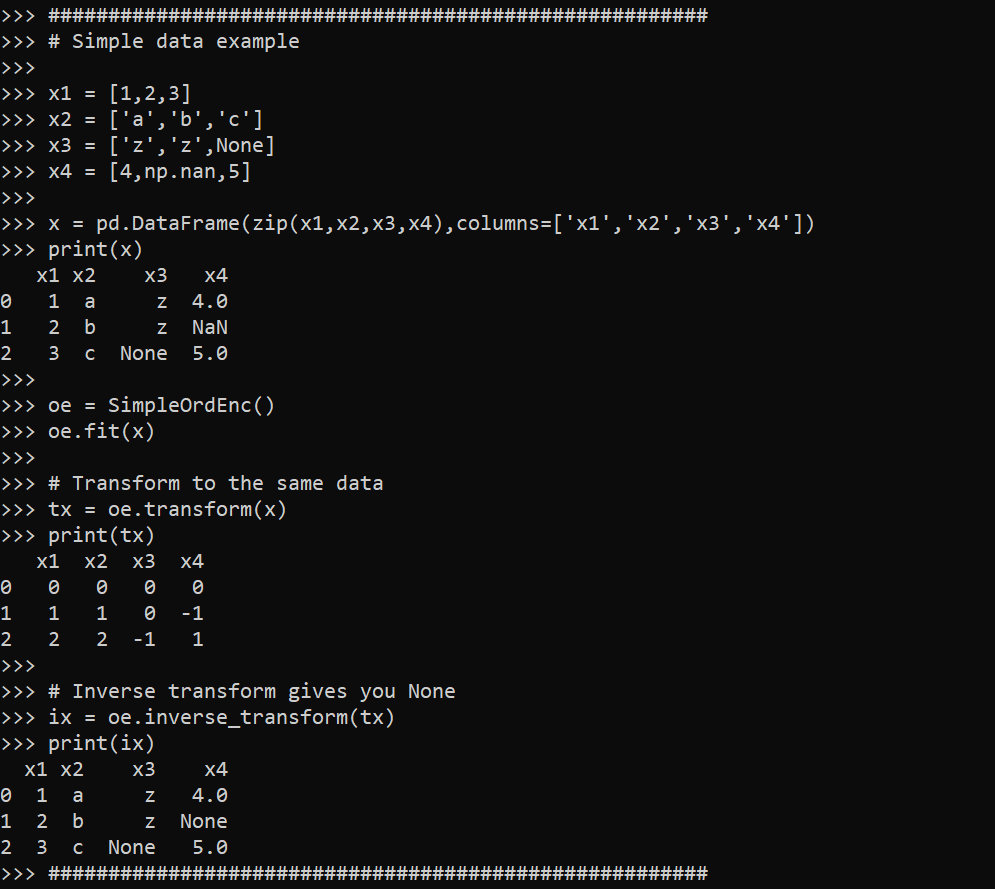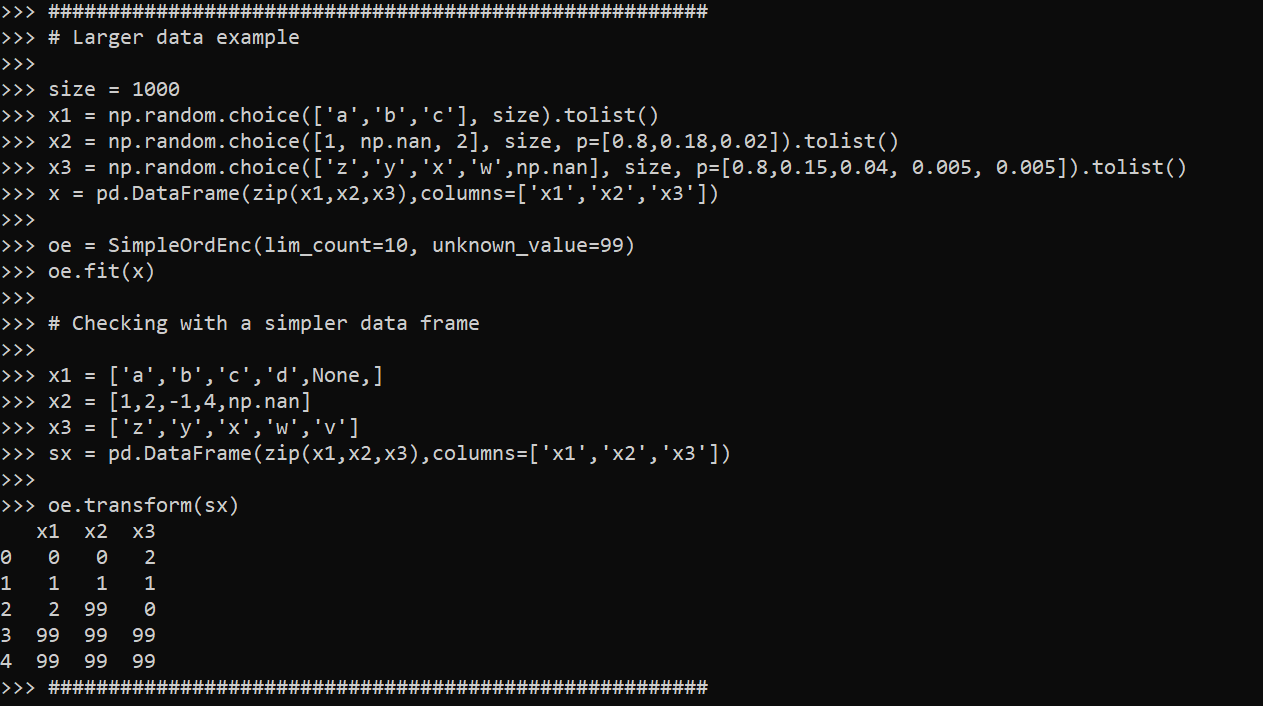# Extending sklearns OrdinalEncoder

I’ve used a variant of this for a few different projects, so figured it was worth sharing. Sklearn’s OrdinalEncoder is close, but not quite what I want for a few different scenarios. Those are:

• mixed input data types
• missing data support (which can vary across the mixed input types)
• the ability to limit encoding of rare categories (useful for regression models)

So I have scripted up a simple new class, what I call `SimpleOrdEnc()`, and can share it here in the blog post.

``````from sklearn.preprocessing import OrdinalEncoder
import numpy as np
import pandas as pd

class SimpleOrdEnc():
def __init__(self, dtype=int, unknown_value=-1, lim_k=None,
lim_count=None):
self.unknown_value = unknown_value
self.dtype = dtype
self.lim_k = lim_k
self.lim_count = lim_count
self.vars = None
self.soe = None
def fit(self, X):
self.vars = list(X)
# Now creating fit for each variable
res_oe = {}
for v in list(X):
res_oe[v] = OrdinalEncoder(dtype=self.dtype,
handle_unknown='use_encoded_value',
unknown_value=self.unknown_value)
# Get unique values minus missing
xc = X[v].value_counts().reset_index()
# If lim_k, only taking top K value
if self.lim_k:
top_k = self.lim_k - 1
un_vals = xc.loc[0:top_k,:]
# If count, using that to filter
elif self.lim_count:
un_vals = xc[xc[v] >= self.lim_count].copy()
# If neither
else:
un_vals = xc
# Now fitting the encoder for one variable
res_oe[v].fit( un_vals[['index']] )
# Appending back to the big class
self.soe = res_oe
# Defining transform/inverse_transform classes
def transform(self, X):
xcop = X[self.vars].copy()
for v in self.vars:
xcop[v] = self.soe[v].transform( X[[v]].fillna(self.unknown_value) )
return xcop
def inverse_transform(self, X):
xcop = X[self.vars].copy()
for v in self.vars:
xcop[v] = self.soe[v].inverse_transform( X[[v]].fillna(self.unknown_value) )
return xcop``````

This works mostly the same way that other sklearn objects do. You instantiate the object, then call fit, transform, inverse_transform, etc. Under the hood it just turns the data into a collection of ordinal encoders, but does a few things. One is that it strips missing values from the original fit data – so out of the box you do not need to do anything like `x.fillna(-1)`, it just works. It is on you though to choose a missing unknown value that does not collide with potential encoded or decoded values. (Also for fit it should be a pandas dataframe, weird stuff will happen if you pass other numpy objects or lists.)

The second are the `lim_k` or the `lim_count` arguments. This is useful if you want to encode rare categories as another value. lim_k is if you want to say keep the top 20 categories in the fitted dataset. lim_count sets the threshold at how many cases are in the data, e.g. if you have at least 100 keep it. lim_k takes precedence over lim_count, so if you specify both lim_count is ignored.

These args also confound the missing values, so missing (even if it is common in the data), gets assiged to the ‘other’ category in this encoding. If that is not the behavior you want, I don’t see any way around not explicitly using `fillna()` before all this.

So here is a simple example use case:

``````x1 = [1,2,3]
x2 = ['a','b','c']
x3 = ['z','z',None]
x4 = [4,np.nan,5]

x = pd.DataFrame(zip(x1,x2,x3,x4),columns=['x1','x2','x3','x4'])
print(x)

oe = SimpleOrdEnc()
oe.fit(x)

# Transform to the same data
tx = oe.transform(x)
print(tx)

# Inverse transform gives you None
ix = oe.inverse_transform(tx)
print(ix)``````So you can see this handles missing input data, but the inverse transform always returns `None` values for missing. The fit method though returns numeric encoded columns with the same variable names. I default to missing values of -1 as light boost (and I think catboost as well), have those as the default missing data values for categorical data.

Here is an example limiting the output to only categories that have at least 10 observations, and setting the missing data value to 99 instead of -1.

``````size = 1000
x1 = np.random.choice(['a','b','c'], size).tolist()
x2 = np.random.choice([1, np.nan, 2], size, p=[0.8,0.18,0.02]).tolist()
x3 = np.random.choice(['z','y','x','w',np.nan], size, p=[0.8,0.15,0.04, 0.005, 0.005]).tolist()
x = pd.DataFrame(zip(x1,x2,x3),columns=['x1','x2','x3'])

oe = SimpleOrdEnc(lim_count=10, unknown_value=99)
oe.fit(x)

# Checking with a simpler data frame

x1 = ['a','b','c','d',None,]
x2 = [1,2,-1,4,np.nan]
x3 = ['z','y','x','w','v']
sx = pd.DataFrame(zip(x1,x2,x3),columns=['x1','x2','x3'])

oe.transform(sx)``````Because the class is all wrapped up in one object, you can then use pickle to save the object/use later in pipelines. If I know I want to test out specific regression models with my data, I often use the lim_count to make sure I am not keeping a bunch of small dangles of high cardinality data. (Often in my data I use missing data is rare enough I don’t even want to worry about imputing, I rather just treat it as a rare category.)

One use case this does not work out so well though for is ICD codes in wide format. Will need to write another blog post about that. But often will just reshape wide to long to fit these encoders.